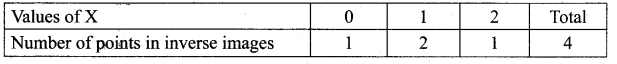## Tamilnadu Samacheer Kalvi 12th Maths Solutions Chapter 11 Probability Distributions Ex 11.1

Question 1.
Suppose X is the number of tails occurred when three fair coins are tossed once simultaneously. Find the values of the random variable X and number of points in its inverse images.
Solution:
Let X is the random variable denotes the number of tails when three coins are tossed simultaneously.
Sample space S = {HHH, HHT, HTH, HTT, THH, THT, TTH, TTT}
∴ ‘X’ takes the values 0,1, 2, 3
i.e., X (HHH) = 0 ; X (HHT, HTH, THH) = 1 ; X (HTT, THT, TTH) = 2 ; X (TTT) = 3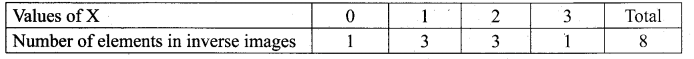Question 2.
In a pack of 52 playing cards, two cards are drawn at random simultaneously. If the number of black cards drawn is a random variable, find the values of the random variable and number of points in its inverse images.
Solution:
Total number of playing cards = 52
Number of Black cards = 26
Number of Non-black (or) Red cards = 26
Let ‘X’ be the random variable denotes the number of black cards. Since two black cards are drawn,’X’ takes the values 0, 1, 2
X (Non-black Cards) = X (26C1 × 25C1) = X (650) = 0
X (1 Black Card) = X (26C1 × 26C0) = X (26) = 1
X (2 Black Cards) = X (26C1 × 25C1) = X (650) = 2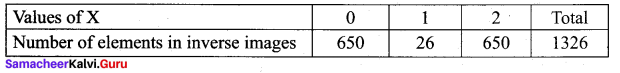Question 3.
An urn contains 5 mangoes and 4 apples. Three fruits are taken at random. If the number of apples taken is a random variable, then find the values of the random variable and number of points in its inverse images.
Solution:
Number of mangoes = 5
Number of Apples = 4
Total number of fruits = 9
Let ‘X’ be the random variable denotes the number of apples taken, then it takes the values 0, 1, 2, 3
X (MMM) = 0
X (AMM (or) MAM (or) MMA) = 1
X (AAM (or) AMA (or) MAA) = 2
X (AAA) = 3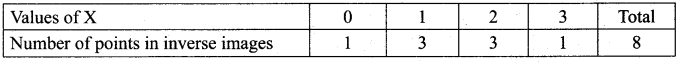Question 4.
Two balls are chosen randomly from an urn containing 6 red and 8 black balls. Suppose that we win ₹ 15 for each red ball selected and we lose ₹ 10 for each black ball selected. X denotes the winning amount, then find the values of X and number of points in its inverse images.
Solution:
Number of red balls = 6
Number of black balls = 8
‘X’ is the random variable denotes the winning amount.
∴ The values of ‘X’ are 0, 15, 30
i.e., X (BB) = 0
X (RB) = 15 + 0 = 15
X (RR) = 15 + 15 = 30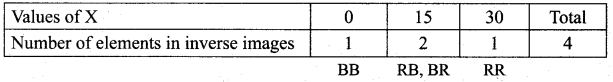Question 5.
A six sided die is marked ‘2’ on one face, ‘3’ on two of its faces, and ‘4’ on remaining three faces. The die is thrown twice. If X denotes the total score in two throws, find the values of the random variable and number of points in its inverse images.
Solution:
Six sided die marked ‘2’ on one face, ‘3’ on two faces and ‘4’ on three faces.
When it is thrown twice, we get 36 sample points.
‘X’ denotes sum of the face numbers and the possible values of ‘X’ are 4, 5, 6, 7 and 8
For X = 4, the sample point is (2, 2)
For X = 5, the sample points are (2, 3), (3, 2)
For X = 6, the sample points are (3, 3), (2, 4), (4, 2)
For X = 7, the sample points are (3, 4), (4, 3)
For X = 8, the sample point is (4, 4)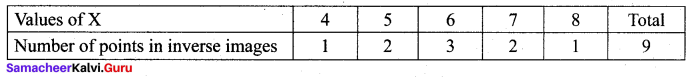### Samacheer Kalvi 12th Maths Solutions Chapter 11 Probability Distributions Ex 11.1 Additional Problems

Question 1.
Four defective oranges are accidentally mixed with sixteen good ones. Two oranges are drawn at random from the mixed lot. If the random variable ‘X’ denotes the number of defective oranges, then find the values of ‘X’ and number of points in its inverse image.
Solution:
Number of good oranges = 16
Number of bad oranges = 4
Total = 20
Let ‘X’ be the random variable denotes the number of bad oranges and it can take the values 0, 1, 2
X (GG) = 0
X (GB (or) BG) = 1
X (BB) = 2